# Project Categories List

## DIY Op Amps

### Introduction

Carl Sagan observed, "If you want to make an apple pie from scratch, you must first create the universe." If you want to build an audio circuit, you will make the decision as to how much you will actually build yourself, and how much you will buy fabricated.

The line is always drawn somewhere. Will you be melting down some sand to make your transistors? Probably not, but it is always in the mind of the hobbyist to do as much as possible. Many of the project articles in AE and elsewhere employ commercially available integrated circuits, usually operational amplifiers.

Monolithic op amps are the gum drops of linear electronics. The Digikey catalog shows hundreds of such parts with prices ranging from about fifty cents to over twenty dollars, depending on performance, package, and manufacturer. Virtually all of these parts would serve in an audio project calling for an op amp.

It is very cheap and easy to use monolithic op amps. In contrast we would suppose that constructing our own op amps from scratch using transistors (or even tubes) would be a daunting task, to be tackled only by seasoned engineers, with no guarantee of satisfaction.

This is not the case at all. Simple high-performance op amps are easy to make out of as few as six discrete components whose total cost is less than a dollar. It is the purpose of this article to show how easy it is.

Besides the satisfaction of doing it yourself, are there any other reasons to construct your own op amps? Yes. First you may want some special characteristic such as very high voltage, or ultra low noise, or high output current, that might be available commercially but at very high cost. Apex, for example, offers high voltage and high current op amps, but you should be prepared to pay as much as \$682 for a PA03A which can deal with 75 volt rails and 30 amp output currents.

For audiophiles with a subjective orientation, there is another reason for building your own op amps which is revealed when you look at the internal schematics of the commercially available chips. Most of them are designed to achieve specifications which are not of great importance to audiophiles, such as ultra high gain, or very low DC drift, or very low dissipation. Their complex topologies reflect these requirements, so you see many transistors and gain stages in series. The output stages of these op amps are operated Class B or AB, so they are not as linear as might be achieved with Class A operation.

We see also that monolithic fabrication techniques do not necessarily deliver the best semiconductor or resistor for each part of the circuit, and by making our own choices in device selection and testing we can achieve high linear performance with very simple circuits. I take it as a

Of course there's really quite a bit more, and most of it involves the concept of feedback. The control pins of the op amp are usually so sensitive that it is nearly impossible to keep the output voltage in the useful (linear) range between the supply rails without some method of controlling the system, and this method is feedback. Feedback works to keep the differential input voltage (the voltage difference between +In and -In) very small, which keep the output in the linear range.We do this by communicating between the negative input and the output of the op amp. The easiest example of this is the simple voltage follower of Fig. 2. In this circuit, the negative input is connected directly to the output, with the result being that the output of the op amp matches the signal presented to the positive input.

Following the logic of an op amp's control pins, we observe that if the voltage from a signal source driving the positive input were to go positive, the output of the op amp would start to go very positive. But the output of the op amp being connected to the negative input would cause it to go positive also, and it will not want to go more positive than the positive input, or else the output would head off in the negative direction. Instead it will come very close to the voltage at the positive input, with just enough difference to allow the op amp's output to track the input.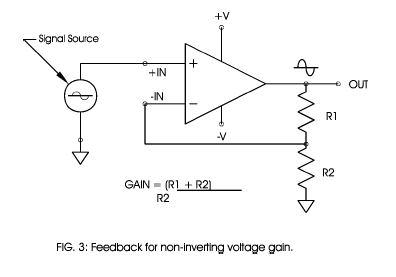#### Gain Devices: A Short Tutorial

Op amps are constructed of gain devices and resistors. The gain devices we will deal with are three terminal devices that are used to control electron flow in a circuit. Current flows through two conducting pins, governed by a third control pin. The voltage or current applied to the control pin is taken relative to one of the other pins. In a Fet or a Tube, the current flowing through the main connections is a function of the control pin voltage relative to one of the other pins, and in a Bipolar transistor, it is a function of the current through the control pin.

In a Fet the control pin is called the Gate. In a Tube it is called the Grid. In a Bipolar transistor it is called the Base. The control exerted by each of these control pins operates relative to one of the other pins in the device. In the Fet the Gate operates relative to the Source pin, controlling the current between the Source and the other pin, the Drain.

In a Tube, the current flows from Plate to Cathode, and is controlled by the voltage between the Grid and the Cathode. In a Bipolar transistor, the current flows from Collector to Emitter, and is controlled by the current between the Base and Emitter.

presumption that simpler circuitry is better, particularly when applied to audio applications.

#### How Op Amps Work

First, a little tutorial on how op amps work. Fig. 1 shows an op amp, which has five connections to the outside world. Two of these are power supply rail pins, +V and -V, which for monolithic op amps usually want to be attached to supply voltages in the range of about 5 to 15 volts each for commercially available chips. Specialized products work outside this range, from as low as 1 volt to as high as 500 volts. There is a presumption here that +V and -V are referenced to a ground potential, but this is not required by the op amp as such. The op amp only needs positive voltage on the +V pin relative to the voltage on the -V pin to operate properly.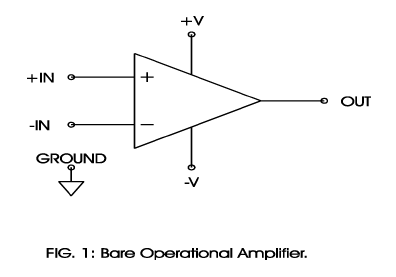An op amp has two input pins, designated the positive and negative inputs (+In and -In), which control the voltage at the output pin. For linear operation we will generally want the voltages appearing at +In and -In to be in the range between the power supply voltages, and in many applications we will see these pins operated near ground potential, midway between +V and -V.

The output of the op amp can vary between +V and -V, and is controlled by the voltage difference between +In and -In. If the voltage at +In is positive with respect to -In, then the output of the op amp swings positive, toward the +V rail voltage. If the voltage at +In is negative compared to the voltage at -In, the output swings negative. It only takes a small difference in voltage between the two inputs to create a large change in the output voltage. This is known as the gain of the op amp.

If we want gain, we simply have to fool the negative input into thinking that the output is smaller than it actually is, as in Fig. 3, where the negative input sees the output voltage after a voltage dividing network. The dividing network reduces the voltage by R2/(R1+R2). As a result, the op amp delivers an output that is a multiple of the input, where the gain is given by the inverse of the divider: (R1+R2)/R2. As an example, if R1 is 9 KOhm and R2 is 1 KOhm, then the gain would be 10, and a 1 volt input at +In would result in a 10 volt output.

Another simple feedback connection allows the negative input to be driven by the signal source, resulting in an inverted output voltage. Fig. 4 shows such a connection, with the gain given by the formula R1/R2. Again following the logic of the op amp input pins, a positive signal from the source will drive the negative input so that the output of the op amp goes very negative, and this feeds back around through R1, greatly reducing the positive voltage. Because in this case the positive input has been tied to ground, the op amp works to keep the negative input very close to ground potential. As a result, this circuit is useful as a mixer, since the negative input can be driven by multiple sources, each with their own resistor, and they won't interact, since the negative input is held to ground potential, a "virtual ground", by the feedback loop of the op amp.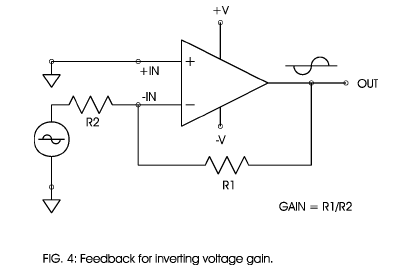A simple rule of thumb about open loop gain and feedback is that the difference between the open loop gain and the actual output gain is how much feedback has been applied. If the open loop gain is 60 dB (X1000) and the actual output gain is 20 dB (X10), then 40 dB (X100) of feedback has been applied.

Certainly there is more to know about feedback, and the above is intended as enough to start playing. There are a number of excellent sources on op amps and feedback, but my favorites come from National Semiconductor's Linear Application Notes ( Digikey (800) 344 4539, order # 9245B-ND ), where 30 years ago I learned most of what I know on the subject. Of particular interest is Bob Widlar's 1968 piece "Monolithic Operational Amplifiers - The Universal Linear Component", as the legendary Widlar is widely regarded as the fountainhead of monolithic op amp design.

It is of interest to know at what rate the current will change respecting the Gate-to- Source voltage. This Fig. is called the transconductance, and tells you how much change in current to expect for a change in Gate voltage. It is often expressed as mhos (the opposite of ohm), because it describes an inverse of resistance. An ohm is volts divided by amps, and a mho is amps divided by volts, so that transconductance is seen as the change of current for a change of control voltage. A device having one mho transconductance will increase its current by 1A for each additional volt on the gate. Most signal Mosfets have transconductance on the order of .1 or so, and power Mosfets over have figures on the order of 10, so that they conduct 10 amps per control volt.

Most N channel Mosfets will begin to conduct somewhere with a gate to source voltage around +3 to +4 volts, and so in linear circuits you will usually see this range of DC voltage between the Gate and Source pins.

Naturally, all this assumes that the Drain of the Mosfet has positive voltage applied to it relative to the Source, otherwise the current won't flow. In much in the same way you can adjust the knob on a water faucet, but you won't get flow unless there is pressure.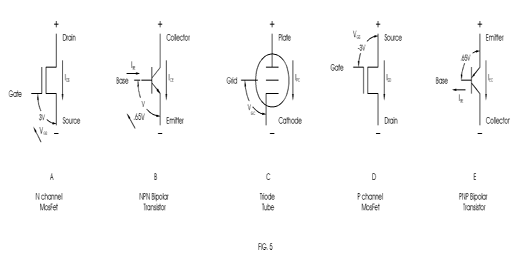Fig. 5 shows these three types of devices, and the voltage and current relationships of their pins. Fig. 5A and 5B show an "N channel" Fet and an NPN transistor, and you will note that 5D and 5E show a "P channel" Fet and a PNP transistor. These "P" types are like the "N" types, but have the current and voltages reversed. The availability of these "negative" versions of the parts is very convenient, and is an advantage over tubes, which have only "N" type polarity.

For the purpose of simplifying this discussion, I will now refer to Mosfets, at the end of which we will make note of the major differences between these types of devices.

As noted, the current flowing through the main pins of the device is a function of the voltage between the control pin and one of the other pins, and in the case of a Mosfet, this would be between the Gate and Source pins. As the voltage on the Gate becomes positive relative to the voltage on the Source, current will begin to flow from the Drain to the Source. This is how the device amplifies electrical signals. The more positive the voltage between the Gate and Source, the more current from the Drain to Source.

For a P channel Mosfet, the action is the same, but the voltages and current flows are reversed. The P channel Mosfet will begin conducting when the Gate to Source voltage reaches around -3 to -4 volts.

The gain devices we will consider have three pins, and there are only three ways to use them. Fig. 6 shows the three ways to use an Nchannel Mosfet, each referred to by a "common pin" name, which is that device pin which does not carry signal voltage. The first use is common Drain operation, also known as follower operation. It has current gain but no voltage gain. The input signal is presented at the Gate, and the output comes from the Source as nearly the same as the input voltage, but shifted - 4 volts DC or so and with a lot more current capacity. The second is common-Source operation, in which we have both voltage and current gain. The input is at the Gate, and a phase inverted output signal appears at the Drain. The load for the transistor is shown as a resistor here, but can be some other, possibly more complex, load.

The third way to use a Mosfet is with common-Gate operation, which has voltage gain but no current gain. Input appears at the Source; output appears at the Drain. This connection is most popularly used to form Cascode operation, in which a common-Gate device shields a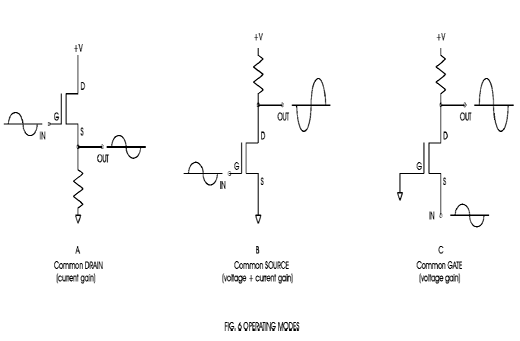common-Source device.

The same principles that govern the use of Mosfets applies to other types of gain devices, but with the following differences:

In JFets, the Gate to Source voltage has a much lower DC value, usually negative, on the order of -1 volt or so. Also, JFets generally have lower transconductance than Mosfets, often on the order of .01 or so.

Tubes require much higher voltages, often greater than 100 volts Plate to Cathode, than solid state devices, and generally conduct less current. For linear operation of a Triode like a 12AX7, the Grid to Cathode DC voltage is a few volts negative, and we see a transconductance on the order of .003, or about 3 milliAmps of current for each control volt.

Bipolar transistors have what you would think of as a very high transconductance Fig., but that is not an appropriate way of looking at them because the current through the device is a function of the current through the control pin. The Base pin voltage relative to the Emitter pin is a relatively constant Fig. on the order of .7 volts, but the current from the Collector to Emitter is a multiple of the Base to Emitter current. This Fig., ranging from less than 10 to as high as 1000, is known as the current gain, or beta, of the Bipolar transistor.

OK, so now we know enough about gain devices to be dangerous, but keep in mind that there are many little details about the characteristics of the various gain devices, details which are generally spelled out in the data sheets and which are worth studying.

#### So let's build some op amps.

Step 1: Constructing a Differential Input

The key to an operational amplifier is the two gain devices that form the differential input. You can build a functional op amp from this alone, although more typically it will be followed by one or more additional stages.

The first step is to construct an input stage known as a differential pair: two transistors with their Sources tied together, and fed current at that connection, as shown in Fig. 7. In this case, current is fed through a current source attached to a negative supply voltage. Two inputs are available at the Gates, as are two outputs at the Drains, which then communicate through loads to the positive supply. For simplicity, the current sources of Fig. 7 are provided by resistors, although a variety of impedance components could be employed. Among the alternatives for these impedances are active constant current sources, where the current will not vary with the voltage. In Fig. 7, I1 is the current sourced to the differential pair which is then evenly divided up into I2 and I3.

The Drains present equal but inverted outputs, which represent the difference between the inputs. If both inputs see the same signal, then that signal ideally does not appear at the outputs. It is a differential amplifier, which means any differences in the input signals at the gates are amplified, and common signal is rejected. For this reason, it is extremely useful as the input stage for an amplifier, because you can connect an input signal to one gate and use the other gate to watch the amplifier output.

This output watching is called feedback. The differential pair is used to compare input and output, and by amplifying their differences it is used to correct for errors at the output.

Depending on the choice of devices, voltages, and resistance values, the differential pair of Fig. 7 will have a certain amount of voltage gain, which in this case is around 20 dB (X10). For some purposes, this might be enough, as in the AE projects "Son of Zen" and "Bride of Son of Zen" in which a power amplifier and accompanying preamplifier are simply differential pairs, nothing more.

Step 2: A Second Gain Stage

Most of the time, though, we want more open loop voltage gain than provided by just a differential pair so we can have some left over for feedback correction. Also, it would be convenient to arrange the circuitry so that the output swings the full voltage of the supplies, which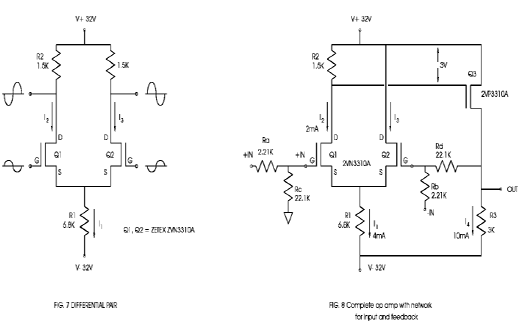the differential pair is not useful. A second gain stage becomes a necessity for this.

Fig. 8 shows the application of a second gain stage to the circuit of Fig. 7. We have added a P channel Mosfet operating in common Source mode and driving a resistor R3 connected to the negative supply. R2 is chosen to give about 3 volts DC to drive the Gate to Source voltage of Q3. This value must often be adjusted to make I2 and I3 equal and create lower offset input voltage for the op amp, since not every P channel Mosfet will want exactly 3 volts of drive.

This is a complete and functional op amp. It has an input CMRR of about -42 dB, and an open loop gain greater than 50 dB. It will swing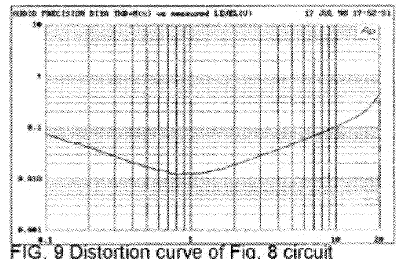20 volts RMS at its output with low distortion, with a slew rate around 80 Volts/microSecond.

Resistors Ra, Rb, Rc, and Rd are not part of the op amp itself. They are an "external" network for input and feedback that make this particular op amp into a linear differential amplifier. Ra is set equal to Rb, and Rc is equal to Rd, and the gain of the amplifier is Rc/Ra. Fig. 9 shows the distortion curve of this particular circuit at 1 KHz between .1 and 20 volts output. Below 1 volt, noise dominates the distortion curve. Measured at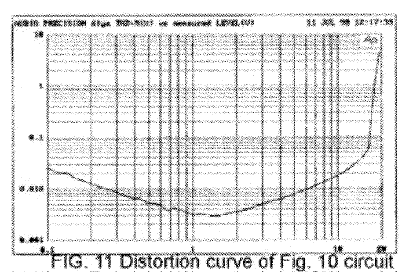2N4250. The Base to Emitter voltages of these devices is consistent at .65 Volts or so, whereas Fets and Tubes are found to have much greater relative differences, and benefit more from matching. You can use Bipolar devices with less gain, commonly around 100 or so, but the performance will decline slightly.

Fig. 12 shows what you might do to create a Triode op amp using 12AX7's. I did not build this device, but I believe it would work adequately for some audio applications, and would offer true DC amplification. The trick of getting the level shifting to properly bias the parallel output tubes lies in creating a DC voltage source interposed between the output plate of the differential pair and the grids of the followers. This can be provided by external DC supplies, such as batteries. This particular circuit would not offer much open loop gain or spectacular performance, but it does illustrate the potential for operational amplifiers without P type gain devices.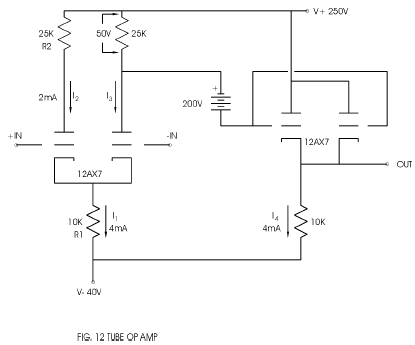Step 3: Still More Gain

The second gain stage of Fig. 8 was created by a P channel device operated in common Source mode, which offers both voltage and current gain. Routinely, this second stage gives us adequate voltage

2 Volts output, the distortion is flat from 20 Hz to 20 KHz. The circuit of 8b is set up for a balanced input, but is easily converted to single input by connecting either of the inputs to ground. Of course you can replace these four resistors with some other network that performs a different task.

We can easily create some other versions of this circuit using other types of gain devices. Fig. 10 shows this circuit rendered with Bipolar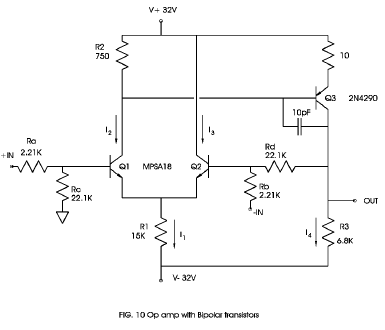transistors rather than Mosfets. It has a higher open loop gain, and yields lower distortion and better CMRR figures than the Mosfet circuit. The distortion curve for this circuit is given in Fig. 11.

Again this is a functional audio gain block that you can build and use. Note that the PNP transistor 2N4250, has an emitter resistor to lower the open loop gain a bit and enhance stability, and that capacitor C1 is employed to frequency stabilize the circuit when operated closed loop.

With Bipolar transistors there is less need to match devices on the differential pair as long as the current gain figures are fairly high. In this case, the MPSA18's have betas of several hundred, as does the

gain for audio applications, but often not enough current gain to drive lower impedance loads. We can achieve more current gain by adding another gain stage operated in common Drain, or follower, mode, which gives us current gain but not voltage gain.

Fig. 13 shows the addition of voltage followers for the circuits of Figs 8 and 10. The open loop output impedance of Figs. 8 and 10 are simply the values of the resistors attached to the negative rail, which is 3 Kohms in Fig. 8 and 6.8 Kohms in Fig. 10. With a closed loop, these values are reduced by feedback, so that 40 dB of feedback would result in functional output impedance of these resistors divided by 100.

The addition of a follower to Fig. 8 results in an open loop output impedance equal to the inverse of the transconductance, resulting in about 20 ohms or so. For the Bipolar device of Fig. 10, it would be the original 6.8 Kohms divided by the current gain (beta) of the transistor, also resulting in about 20 ohms or so.

The follower not only gives a lower output impedance, but makes the performance of the op amp less dependent on the load impedance. If you want to drive a lower impedance load, particularly a loudspeaker, you will want some current gain, and very often you will want this third stage.

Keep in mind that these issues heavily depend on the requirements of the circuit and the type of device. As a practical matter, Mosfets give the best combination of characteristics for performance from the most simple power circuits at higher currents, but JFets are superior at low power levels for noise and input impedance. Bipolar signal devices give very high gain in low impedance circuits, and so they often measure better in cases where the source impedance is low.

Step 4: More Performance

Constant Current Sources

So far we have built these op amps using resistors to set up the currents (bias) through the gain devices. This is a simple and linear way to do it, but often we want to bias the gain devices with currents that do not vary with voltage, as they will with resistors. These are called constant current sources. Constant current sources operate so as to pass a constant current over a wide range of voltages, and in so doing, they can improve the environment in which the gain devices work. Fig. 14 shows the Mosfet and Bipolar op amps of Fig. 13 where the resistors have been replaced by constant current sources.

Fig. 15a shows the details of these current sources as made with Mosfets. It takes about 3.5 volts to bias up the Gate to Source pins of a Mosfet, so we create a voltage reference using the Zener diode and resistor. The resistor runs a small amount of current through the Zener diode, which creates a constant voltage. We use this voltage, 9.1 volts, to drive the Gate of the Mosfet. The Gate to Source requirements will eat up 3.5 volts of this, leaving 5.5 volts across the Source resistor, which causes the Drain of the Mosfet to source current as determined by 5.5/R. The 221 ohm resistor in series with the Gate prevents parasitic oscillation in the Mosfet, and is generally essential. This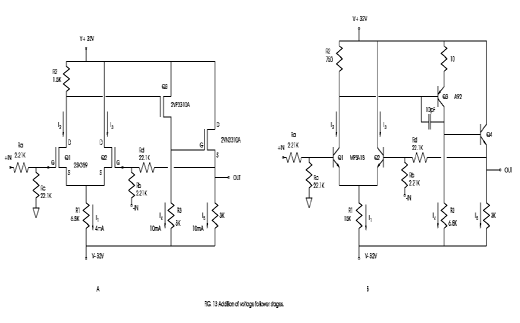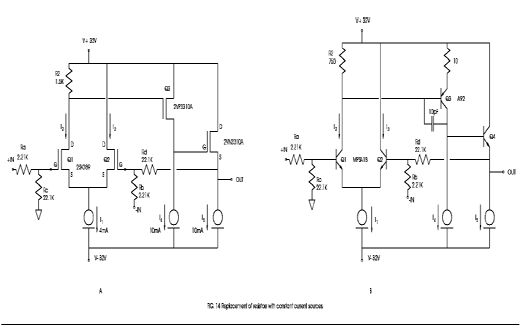current source works quite well, and can be easily used at high voltages and high currents. It tends to be noisy at low level usage, but this can be improved by placing a capacitor across the Zener diode, which is a major contributor to the random noise.

Fig. 15b shows the current sources as made with Bipolar devices. In this case, the bipolar transistor forming the current source is driven by bias voltage created using a pair of diodes. The diodes drive the base of the transistor with about 1.3 volts, and the Base to Emitter junction drops about half of that, leaving about .65 volts left over to determine the current through the Emitter resistor of the current source. The current coming out of the Collector will be relatively constant at .65/R.

Fig. 15c shows current sources formed from JFets. JFets normally have a negative gate voltage, and so they conveniently form a self-biased current source as shown. For an N channel device like the 2SK170, the gate voltage is about -.3 volts, so we simply set the source resistor value for current determined by .3/R. As shown, the current sources are set for 4 milliAmps. Besides simplicity, JFets have the advantage of being very low noise. On the down side, they are limited in their ability to handle high voltages and dissipate wattage.

The choice of which device to use for current sources is up to you. There is not requirement that you use Mosfets current sources in the Mosfet op amp, nor is there are requirements that you must use current sources everywhere in the circuit. You are free to use current sources where you choose, or not, depending on the results you want.

The willingness to experiment is very important to getting the results you want. You can evaluate performance subjectively or objectively, but you should always be prepared to try things out.

Using a constant current source to bias the differential pair (I1) is probably the most key improvement. It has the advantage that it does not raise the open loop gain, but dramatically improves the power supply rejection (PSRR), making the performance independent of supply fluctuation and noise. It also greatly improves the common mode rejection (CMRR) of the input stage, typically by a factor of 20 to 30 dB.

The second most useful spot for a constant current source is where the second gain stage is loaded (I4). This raises the gain of this stage, lowers the distortion, and again improves the power supply rejection.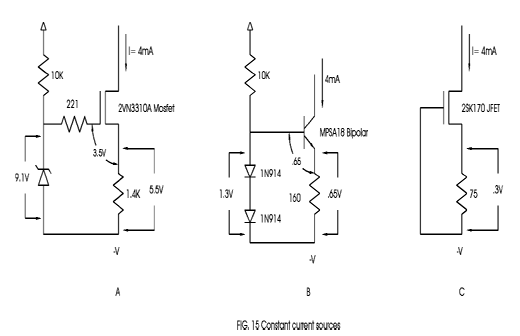I have not shown current sourcing where R2 is replaced by a constant current source, and this is a technique often used in monolithic op amps as it increases the gain of the differential pair and raises the open loop gain of the op amp, sometimes dramatically. Sometimes it is necessary when you need very high gain, such as in phono stages, but it is often more trouble to implement than it is worth in line level applications. The requirement for low voltage across this current source makes it a little more difficult, and we will leave it as a future advanced exercise.

Current sourcing the third output follower stage (I5) gives some linearity improvement and improves the efficiency and PSRR of a single-ended Class A output stage, but is often not essential to the performance of a line level stage with a regulated supply.

#### Lowering the Noise

Part of having low noise is either having high power supply rejection (PSRR) or a quiet power supply. It is not difficult to use quiet current sources or a quiet power supply or both. After that, the noise will depend heavily on the quality of the input transistors.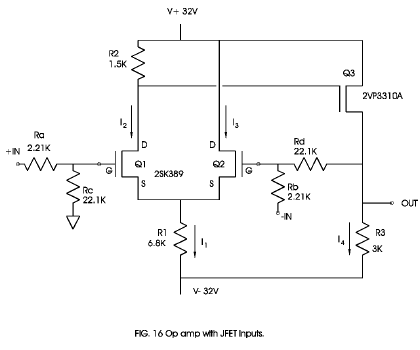find yourself wanting to transplant some of our example circuits into these projects, in which case you have the choice of either creating higher voltage supplies or adjusting the biasing of the op amps.

As you raise the supply rails, the values bias resistors R1, R3, and R4 are increased to give the same current, and at higher values they look more like constant current sources to a given level of signal. This is a very easy way to improve the performance without introducing more parts.

If you choose to use the lower 15 Volt rails of an existing project, you will want to adjust the resistors which determine I1, I4, and I5. As a practical matter, you can simply halve their values to get the same amount of current, and the reduction in performance will be nominal, but not likely a real problem. You will not be halving the value of R2 as long as I1 remains the same.

None of these bias values are critical; in fact I pulled them out of the air without any attempt to optimize the performance. If you play around with the various values of resistance and current, you may find the performance improved. If you have a distortion analyzer, it is perfectly acceptable to tweak the values for a lower distortion number.

It is highly probable that you will want to adjust the resistors in a particular application to get low DC offset at the output, so don't be afraid to play around. Remember, none of these parts costs as much as a dollar.

Likewise, feel free to substitute different kinds of gain devices in and out. The circuits given here will work with just about any kind of part, and you can compensate for many differences simply by adjusting R2.

There will be times when your circuits will oscillate, which is one of the best reasons for owning an oscilloscope. Oscillation, in which the feedback loop chases its own tail, causes a lot of distortion and noise. There are several things you can do to eliminate or reduce it:

First, bypass the power supply leads to ground with capacitors near the circuit.

Second, always place some resistance (a couple hundred ohms or so) in series with the gate of any Mosfet.

Third, never hook the output of a small current op amp to the outside world without some resistance in series with the output, at least 100 ohms or so.

Fourth, use compensation capacitors in the circuit such as C1 in Fig. 10, and don't be afraid to adjust their values.

Fifth, place resistance in series with the Source or Emitter pins of gain devices Q1, Q2, and Q3 to reduce their gain. If you use resistance on Q1 of the differential pair, then use the same value on Q2 to keep them equal.

Generally we want input impedance to be high, so that it doesn't load down or otherwise distort the source, but there is a category of op amp using "current feedback" in which the input impedance of the negative input is low. If you need or want high input impedance, JFets give the best performance, followed by Mosfets and then by Bipolar transistors.

Input Bias Current: If the input pins are attached to bipolar transistors, there will be a small but measurable DC current flowing through the input connections which might be important in some applications. JFets and Mosfets do no suffer from this in any practical sense.

Common Mode Input Rejection Ratio: While only the differential input voltage is what is supposed to drive the op amp's output, we find that the voltage which is common to both inputs can have an effect too. The amount of this effect is abbreviated as the CMRR, and is given in decibels. An op amp with a CMRR of -60 dB and an open loop gain of 60 dB will have 1 Volt of output or each volt of common mode input signal. If 40 dB of feedback is used to reduce the gain to 20 dB, then it will have .01 Volt output for each volt of input common mode.

Power Supply Rejection Ratio: The ideal op amp does not depend on the value of the supply voltages, nor does any supply noise appear at the output. As a practical matter, this will not be the case, and like CMRR, this is expressed as in dB. Monolithic op amps usually have very high PSRR Fig.s. Home brew op amps typically are not as good, giving an excuse for having quiet regulated supplies.

Power Supply Range: The functional range of +V and -V. Traditionally, projects will choose 15 volt supply rails. For line level audio applications, this is often adequate, however we might want higher voltages for use in power amplifiers, or to achieve greater linearity from the gain devices used in the op amp, but this will be limited by the ratings of the gain devices.

Output Impedance: Just as the input pins have a finite impedance, so does the output pin, only here we want the impedance to be low. Often it is not actually very low, but is made effectively low by the action of the feedback loop. For example, an op amp whose intrinsic "open loop" output impedance is 10 ohms and whose open loop gain is 1 million (120 dB), will operate as a follower with an output impedance of 10 millionths of an ohm. A follower stage at the output of the op amp is routinely use to deliver a low output impedance, if needed.

Output Current: Output current is limited by the gain and dissipation capacity of the op amp's output stage, and for monolithic devices, this is on the order of 10 milliamps (.01 amp) or so. Audio power amplifiers are usually just big op amps whose output current ratings are several amps, sometimes hundred of amps.

Bandwidth: The op amp's open loop gain will decline , or roll off, with frequency, and the frequency at which this begins to occur is the bandwidth of the op amp. Many monolithic op amps with very high gain will experience this roll off below audio frequencies, such as 10 Hz. This is not necessarily the response of the op amp with a feedback loop, as the feedback offers correction, however the ability of the feedback to correct for declining open loop declines at the same rate.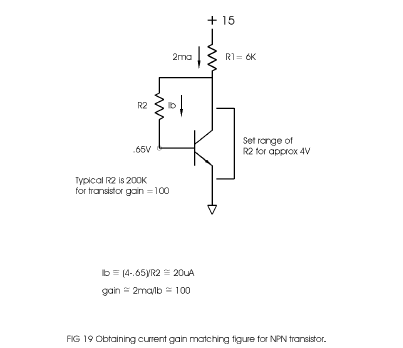transistors with a current gain of 100, the resistor will be about 200 KOhms, allowing current of about 20 microAmps to flow through the Base and causing 2 milliAmps to flow through the transistor. For higher gain devices, higher values of base resistance will be appropriate, chosen to place about 4 Volts or so across the transistor. As before, we match devices for the same voltage across the device given the same Base resistor.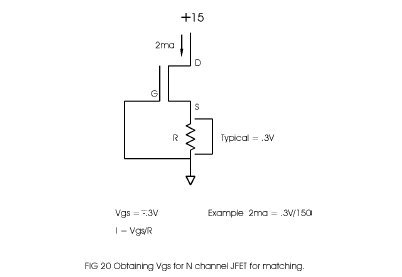Fig. 20 shows the matching circuit for N channel JFets. Because JFets do not require a positive control voltage, they are conveniently selfbiasing in this test. Choose the value of the resistor so that the typical JFet operates at 2 milliAmps, as determined by the voltage across the resistor and the value of the resistor, where I = V/R. For example, if the voltage is .3 Volts, then .002 = .3 / R, which results in an R of 150 Ohms. In this case we measure the voltage across this resistor, not R1, and we want our matched devices to have the same voltage.

We note on the distortion curves of our op amps that the distortion curve is seen to rise as the output goes below a volt or so. This is mostly due to random noise in the differential input devices. For the Mosfet circuit of Fig. 8, this amounts to about 10 microVolts of noise. For the Bipolar circuit of Fig. 10 it is about 2 microVolts.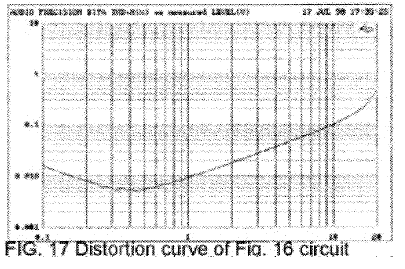For even lower noise, it is possible to use low noise JFets as the differential pair, giving random input noise on the order of .4 microVolts. Fig. 16 shows the circuit of Fig. 8 but with 2SK389 dual low noise JFets dropped in. The distortion and noise at low levels drops by an order of magnitude, but the performance at higher levels is nearly identical, as seen in Fig. 17.

#### Miscellaneous

All of the example op amps presented here have used 32 Volt rails, which represents a reasonably good trade off between performance of the simple circuits and the ratings of the devices. We can get some interesting improvements at higher voltages with higher voltage devices and use of cascode operation, but that is beyond the scope of what we are trying to accomplish here.

Projects using monolithic op amps routinely have plus and minus 15 volt rails, as this represents the supply ratings of many devices. You may

#### Conclusion

This article has been intended only as a beginning tutorial, something to get you started playing with and building your own op amps. Going through the literature on op amp schematics, applications, and characteristics of gain devices, you will see that we have just scratched the surface. Nevertheless, you can take the circuits presented here and put them to use directly or extend them in ways limited only by your imagination and willingness to experiment. Fooling around with this stuff is how you learn.

At our web site, www.passlabs.com, you will find copies of other DIY projects which will help by providing more tutorial discussions and more examples of op amps put to work. You can e-mail me from there, or more directly: nelson@passlabs.com . Sooner or later everyone gets a reply, sometimes the one desired.

I get a lot of mail asking for the names of books that will teach you how to build amplifiers, and I don't have a good response. As mentioned before, the National Semiconductor Linear App Notes is a great source of information. Also, "The Art of Electronics", by Horowitz and Hill, Cambridge University Press, is an excellent book that I keep around. Both of these sources have more information than you are likely to want.

#### Sidebar: Op Amp Specifications

There are a number of useful specifications regarding operational amplifiers, whether they are monolithic chips or do-it-yourself discretes. Here is a quick glossary of the most commonly quoted specs. Remember that decibels (dB) is a nonlinear scale for describing the ratio of two numbers, and that every factor of 10 adds 20 dB.

Performance specifications are the results of the laws of nature and the decisions made by the designer. Typically you have to give up something somewhere to get something else, so it is always appropriate to consider what specifications are important to you, and what numbers are appropriate, and what you give up to get them. If one design delivered it all, then there would only be one design. From the catalogs, I would guess that there are thousands of designs.

Open Loop Gain: The ratio of output voltage change over differential input voltage change. If a difference of .001 volt between the positive and negative inputs results in 1 volt of output, the gain is 1000, or 60 dB. Most op amps are designed for very high gain, often on the order of 1 million (120 dB), but this is not always necessary or desirable, as you often trade off complexity and bandwidth, and when you design your own op amps you can adjust the gain to your needs.

Input Offset Voltage: You can imagine that if the differential inputs of the op amp were perfectly matched, then the output of the op amp would settle to the midpoint between the supply rails when the differential input voltage is 0. Typically it does not, and the slight input voltage required to place the output between the supply rails, typically measured in milliVolts, is known as the input offset voltage.

Input Impedance: The input pins of an op amp have a finite impedance, and will draw some small amount of current due to an input voltage.

Slew Rate: Closely related to the bandwidth, the question is "How fast can the output move from one voltage to another?" Monolithic devices have speeds anywhere from less than 1 volt per microsecond to over 1000 volts per microsecond. Line level audio circuits experience signal speeds as high as .1 volts per microsecond with music, and the project circuits here all do around 80 volts per microsecond or more.

Distortion: All circuits distort the signal, and the question is simply how much. The most common specification is Total Harmonic Distortion plus Noise (THD+N) which you see in the distortion curves here. When a simple single frequency signal (a sine wave) is distorted by a circuit, additional components appear at multiples, or harmonics, of the original frequency. Adding up the energy in these harmonics plus the noise gives a percentage number. Obviously low distortion and noise is better but such emphasis has been placed on this single number that many audiophiles are concerned that some other performance factor has been traded off against this. There is a common belief among audiophiles that Distortion does not tell the whole story.

#### Sidebar: Matching Differential Gain Devices

The best performance is obtained from differential pairs where the devices are matched. You can often buy them this way on a single chip, but it is not difficult to do some simple matching yourself. The test is simple and requires a power supply, a resistor, and a DC voltmeter.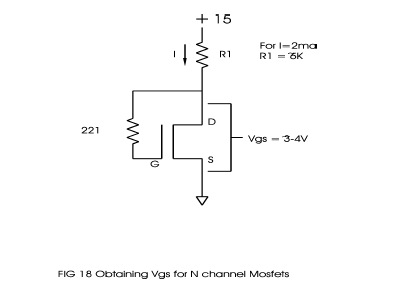Fig. 18 shows the test hookup for N channel Mosfets. The supply source resistance (R1) is nominal, and is found from I2 = (V - 3)/R1, where V is the supply voltage and I is the nominal current you intend to run each input device at. In the op amp circuits presented here, I2 is about 2 milliAmps. Given a 15 Volt supply, R1 should be about 6000 ohms.

We are looking to match the voltage across the Mosfet, which will be around 3 to 4 Volts. Keep in mind the caveats about electrostatic discharge: touch ground before you touch the parts.

Fig. 19 shows the matching circuit for NPN transistors. We use the same R1 value, but the resistor in series with the Base should be chosen so that a typical part has about 4 Volts across Collector to Emitter. For